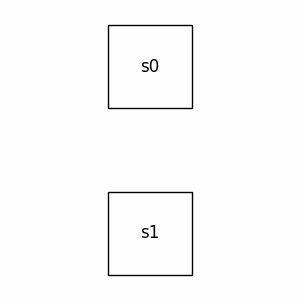# monoidal#

The free (pre)monoidal category, i.e. planar diagrams.

## Summary#

 `Ty` A type is a tuple of objects with `Ty.tensor()` as concatenation. `PRO` A PRO is a natural number `n` seen as a type with addition as tensor. `Layer` A layer is a `box` in the middle of a pair of types `left` and `right`. `Diagram` A diagram is a tuple of composable layers `inside` with a pair of types `dom` and `cod` as domain and codomain. `Box` A box is a diagram with a `name` and the layer of just itself inside. `Sum` A sum is a tuple of diagrams `terms` with the same domain and codomain. `Bubble` A bubble is a box with a diagram `arg` inside and an optional pair of types `dom` and `cod`. `Category` A monoidal category is a category with a method `tensor`. `Functor` A monoidal functor is a functor that preserves the tensor product. `Whiskerable` Abstract class implementing the syntactic sugar `@` for whiskering and parallel composition with some method `tensor`.

## Axioms#

We can check the axioms for `Ty` being a monoid.

```>>> x, y, z, unit = Ty('x'), Ty('y'), Ty('z'), Ty()
>>> assert x @ unit == x == unit @ x
>>> assert (x @ y) @ z == x @ y @ z == x @ (y @ z)
```

We can check the axioms for dagger monoidal categories, up to interchanger.

```>>> x, y, z, w = Ty('x'), Ty('y'), Ty('z'), Ty('w')
>>> f0, f1 = Box('f0', x, y), Box('f1', z, w)
>>> d = Id(x) @ f1 >> f0 @ Id(w)
>>> assert d == (f0 @ f1).interchange(0, 1)
>>> assert f0 @ f1 == d.interchange(0, 1)
>>> assert (f0 @ f1)[::-1][::-1] == f0 @ f1
>>> assert (f0 @ f1)[::-1].interchange(0, 1) == f0[::-1] @ f1[::-1]
```

We can check the Eckmann-Hilton argument, up to interchanger.

```>>> s0, s1 = Box('s0', Ty(), Ty()), Box('s1', Ty(), Ty())
>>> assert s0 @ s1 == s0 >> s1 == (s1 @ s0).interchange(0, 1)
>>> assert s1 @ s0 == s1 >> s0 == (s0 @ s1).interchange(0, 1)
```